# Set Functions¶

class improb.setfunction.SetFunction(pspace, data=None, number_type=None)

A real-valued set function defined on the power set of a possibility space.

__init__(pspace, data=None, number_type=None)

Construct a set function on the power set of the given possibility space.

Parameters: pspace (list or similar; see Possibility Spaces) – The possibility space. data (dict) – A mapping that defines the value on each event (missing values default to zero).
__repr__()
>>> SetFunction(pspace=3, data={(): 1, (0, 2): 2.1, (0, 1, 2): '1/3'})
SetFunction(pspace=PSpace(3),
data={(): 1.0,
(0, 2): 2.1,
(0, 1, 2): 0.333...},
number_type='float')
>>> SetFunction(pspace=3, data={(): '1.0', (0, 2): '2.1', (0, 1, 2): '1/3'})
SetFunction(pspace=PSpace(3),
data={(): 1,
(0, 2): '21/10',
(0, 1, 2): '1/3'},
number_type='fraction')

__str__()
>>> print(SetFunction(pspace='abc', data={'': '1', 'ac': '2', 'abc': '3.1'}))
: 1
a   c : 2
a b c : 31/10

get_bba_choquet(gamble)

Calculate the Choquet integral of the set function as a basic belief assignment.

Parameters: gamble – dict or similar; see Gambles

The Choquet integral of a set function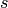is given by the formula: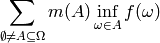where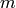is the Mobius transform of.

Warning

In general, improb.setfunction.SetFunction.get_choquet() is far more efficient.

improb.lowprev.lowprob.LowProb.is_completely_monotone()
To check for complete monotonicity.
improb.setfunction.SetFunction.get_mobius()
Mobius transform of an arbitrary set function.
get_choquet(gamble)

Calculate the Choquet integral of the given gamble.

Parameters: gamble – dict or similar; see Gambles

The Choquet integral of a set functionis given by the formula: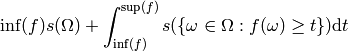for any gamble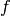(note that it is usually assumed that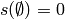). For the discrete case dealt with here, this becomes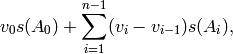where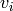are the unique values ofsorted in increasing order and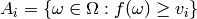are the level sets induced.

>>> s = SetFunction(pspace='abc', data={'': 0,
...                                     'a': 0, 'b': 0, 'c': 0,
...                                     'ab': .5, 'bc': .5, 'ca': .5,
...                                     'abc': 1})
>>> s.get_choquet([1, 2, 3])
1.5
>>> s.get_choquet([1, 2, 2])
1.5
>>> s.get_choquet([1, 2, 1])
1.0


Warning

The set function must be defined for all level sets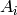induced by the argument gamble.

>>> s = SetFunction(pspace='abc', data={'ab': .5, 'bc': .5, 'ca': .5,
...                                     'abc': 1})
>>> s.get_choquet([1, 2, 2])
1.5
>>> s.get_choquet([2, 2, 1])
1.5
>>> s.get_choquet([-1, -1, -2])
-1.5
>>> s.get_choquet([1, 2, 3])
Traceback (most recent call last):
...
KeyError: Event(pspace=PSpace(['a', 'b', 'c']), elements=set(['c']))

classmethod get_constraints_bba_n_monotone(pspace, monotonicity=None)

Yields constraints for basic belief assignments with given monotonicity.

Parameters: pspace (list or similar; see Possibility Spaces) – The possibility space. monotonicity (int or collections.Iterable of int) – Requested level of monotonicity (see notes below for details).

This follows the algorithm described in Proposition 2 (for 1-monotonicity) and Proposition 4 (for n-monotonicity) of Chateauneuf and Jaffray, 1989. Some characterizations of lower probabilities and other monotone capacities through the use of Mobius inversion. Mathematical Social Sciences 17(3), pages 263-283:

A set functiondefined on the power set of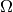is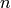-monotone if and only if its Mobius transformsatisfies: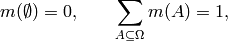and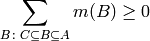for all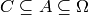, with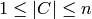.

This implementation iterates over all, with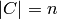, and yields each constraint as an iterable of the events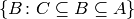. For example, you can then check the constraint by summing over this iterable.

Note

As just mentioned, this method returns the constraints corresponding to the latter equation for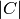equal to monotonicity. To get all the constraints for n-monotonicity, call this method with monotonicity=xrange(1, n + 1).

The rationale for this approach is that, in case you already know that (n-1)-monotonicity is satisfied, then you only need the constraints for monotonicity=n to check for n-monotonicity.

Note

The trivial constraints that the empty set must have mass zero, and that the masses must sum to one, are not included: so for monotonicity=0 this method returns an empty iterator.

>>> pspace = "abc"
>>> for mono in xrange(1, len(pspace) + 1):
...     print("{0} monotonicity:".format(mono))
...     print(" ".join("{0:<{1}}".format("".join(i for i in event), len(pspace))
...                    for event in PSpace(pspace).subsets()))
...     constraints = SetFunction.get_constraints_bba_n_monotone(pspace, mono)
...     constraints = [set(constraint) for constraint in constraints]
...     constraints = [[1 if event in constraint else 0
...                     for event in PSpace(pspace).subsets()]
...                    for constraint in constraints]
...     for constraint in sorted(constraints):
...         print(" ".join("{0:<{1}}"
...                        .format(value, len(pspace))
...                        for value in constraint))
1 monotonicity:
a   b   c   ab  ac  bc  abc
0   0   0   1   0   0   0   0
0   0   0   1   0   0   1   0
0   0   0   1   0   1   0   0
0   0   0   1   0   1   1   1
0   0   1   0   0   0   0   0
0   0   1   0   0   0   1   0
0   0   1   0   1   0   0   0
0   0   1   0   1   0   1   1
0   1   0   0   0   0   0   0
0   1   0   0   0   1   0   0
0   1   0   0   1   0   0   0
0   1   0   0   1   1   0   1
2 monotonicity:
a   b   c   ab  ac  bc  abc
0   0   0   0   0   0   1   0
0   0   0   0   0   0   1   1
0   0   0   0   0   1   0   0
0   0   0   0   0   1   0   1
0   0   0   0   1   0   0   0
0   0   0   0   1   0   0   1
3 monotonicity:
a   b   c   ab  ac  bc  abc
0   0   0   0   0   0   0   1

get_mobius(event)

Calculate the value of the Mobius transform of the given event. The Mobius transform of a set functionis given by the formula: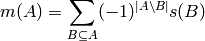for any event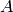.

Warning

The set function must be defined for all subsets of the given event.

>>> setfunc = SetFunction(pspace='ab', data={'': 0, 'a': 0.25, 'b': 0.3, 'ab': 1})
>>> print(setfunc)
: 0.0
a   : 0.25
b : 0.3
a b : 1.0
>>> inv = SetFunction(pspace='ab',
...                   data=dict((event, setfunc.get_mobius(event))
...                        for event in setfunc.pspace.subsets()))
>>> print(inv)
: 0.0
a   : 0.25
b : 0.3
a b : 0.45

get_zeta(event)

Calculate the value of the zeta transform (inverse Mobius transform) of the given event. The zeta transform of a set functionis given by the formula: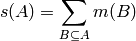for any event(note that it is usually assumed that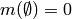).

Warning

The set function must be defined for all subsets of the given event.

>>> setfunc = SetFunction(
...     pspace='ab',
...     data={'': 0, 'a': 0.25, 'b': 0.3, 'ab': 0.45})
>>> print(setfunc)
: 0.0
a   : 0.25
b : 0.3
a b : 0.45
>>> inv = SetFunction(pspace='ab',
...                   data=dict((event, setfunc.get_zeta(event))
...                             for event in setfunc.pspace.subsets()))
>>> print(inv)
: 0.0
a   : 0.25
b : 0.3
a b : 1.0

is_bba_n_monotone(monotonicity=None)

Is the set function, as basic belief assignment, n-monotone, given that it is (n-1)-monotone?

Note

To check for n-monotonicity, call this method with monotonicity=xrange(n + 1).

Note

For convenience, 0-montonicity is defined as empty set and possibility space having lower probability 0 and 1 respectively.

Warning

The set function must be defined for all events.

classmethod make_extreme_bba_n_monotone(pspace, monotonicity=None)

Yield extreme basic belief assignments with given monotonicity.

Warning

Currently this doesn’t work very well except for the cases below.

>>> bbas = list(SetFunction.make_extreme_bba_n_monotone('abc', monotonicity=2))
>>> len(bbas)
8
>>> all(bba.is_bba_n_monotone(2) for bba in bbas)
True
>>> all(bba.is_bba_n_monotone(3) for bba in bbas)
False
>>> bbas = list(SetFunction.make_extreme_bba_n_monotone('abc', monotonicity=3))
>>> len(bbas)
7
>>> all(bba.is_bba_n_monotone(2) for bba in bbas)
True
>>> all(bba.is_bba_n_monotone(3) for bba in bbas)
True
>>> bbas = list(SetFunction.make_extreme_bba_n_monotone('abcd', monotonicity=2))
>>> len(bbas)
41
>>> all(bba.is_bba_n_monotone(2) for bba in bbas)
True
>>> all(bba.is_bba_n_monotone(3) for bba in bbas)
False
>>> all(bba.is_bba_n_monotone(4) for bba in bbas)
False
>>> bbas = list(SetFunction.make_extreme_bba_n_monotone('abcd', monotonicity=3))
>>> len(bbas)
16
>>> all(bba.is_bba_n_monotone(2) for bba in bbas)
True
>>> all(bba.is_bba_n_monotone(3) for bba in bbas)
True
>>> all(bba.is_bba_n_monotone(4) for bba in bbas)
False
>>> bbas = list(SetFunction.make_extreme_bba_n_monotone('abcd', monotonicity=4))
>>> len(bbas)
15
>>> all(bba.is_bba_n_monotone(2) for bba in bbas)
True
>>> all(bba.is_bba_n_monotone(3) for bba in bbas)
True
>>> all(bba.is_bba_n_monotone(4) for bba in bbas)
True
>>> # cddlib hangs on larger possibility spaces
>>> #bbas = list(SetFunction.make_extreme_bba_n_monotone('abcde', monotonicity=2))

pspace

An PSpace representing the possibility space.

More Examples

Decision Making